# RBI Assistant Pre 2023 Quantitative Aptitude Quiz -27

## RBI Assistant Pre 2023 Quantitative Aptitude

Quantitative Aptitude is the most significant part of almost all competitive exams. Many candidates find difficulty in solving Quantitative Aptitude Questions. The only way to ace this section is to practice as many questions as possible. And, to help you practice more and more questions, we have come up with the RBI Assistant Pre 2023 Quantitative Aptitude Quiz. This RBI Assistant Pre 2023 Quantitative Aptitude Quiz is completely Free. This RBI Assistant Pre 2023 Quantitative Aptitude Quiz includes a variety of questions that are asked in exams. Candidates will be provided with a detailed solution for each question in this RBI Assistant Pre 2023 Quantitative Aptitude Quiz. This RBI Assistant Pre 2023 Quantitative Aptitude Quiz will assist candidates in better preparing for upcoming exams.

Directions (1– 5): Study the given table carefully and answer the questions.

Table shows the total population in six different cities and the ratio of literate to illiterate population and also the   percentage of graduate out of literate population in each city.1. Graduate population of city B and D together is approximately what percent more/less than graduate population of city A and E together?

(a) 54%

(b) 50%

(c) 47%

(d) 42%

(e) 37%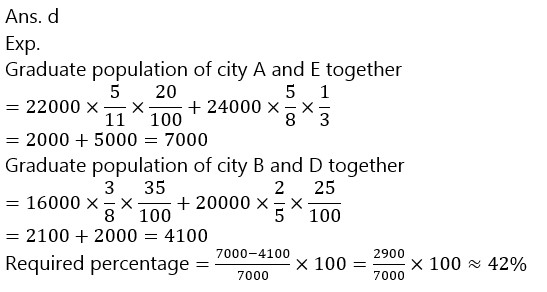1. Population of city C who are literate but not graduate is how much more than the average graduate population of city D and E together?

(a) 40020

(b) 4020

(c) 4200

(d) 4420

(e) 400401. If the ratio of illiterate male to female in city B is 3:5 and ratio of graduate male to female population in city D is 2 : 3. Then find the ratio of total illiterate male in city B and graduate female in city D?

(a) 23 : 7

(b) 8 : 25

(c) 75 : 16

(d) 21 : 8

(e) 25 : 8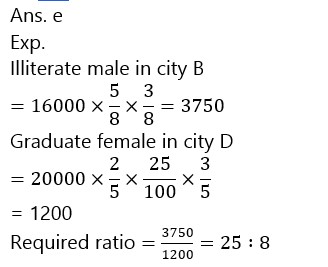1. Illiterate population of city D is what percent of the Illiterate population of city ‘C’?

(a) 25%

(b) 37.5%

(c) 40%

(d) 50%

(e) 62.5%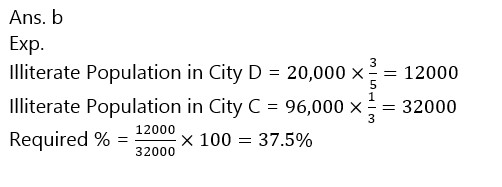1. Literate population of cities A and B together is approximately what percentage of the population which are not graduate of city D?

(a) 82%

(b) 72%

(c) 93%

(d) 79%

(e) 89%Directions (6-10): In each of these questions, two equations (I) and (II) are given. You have to solve both the equations and give answer(a)        if x>y

(b)        if x≥y

(c)         if x<y

(d)        if x ≤y

(e)        if x = y or  no relation can be established between x and y.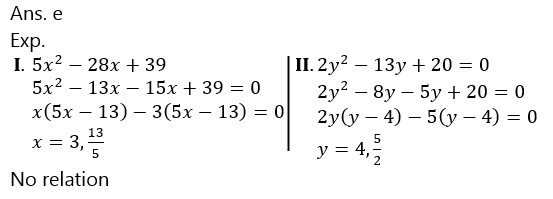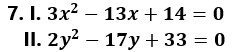(a)        if x>y

(b)        if x≥y

(c)         if x<y

(d)        if x ≤y

(e)        if x = y or  no relation can be established between x and y.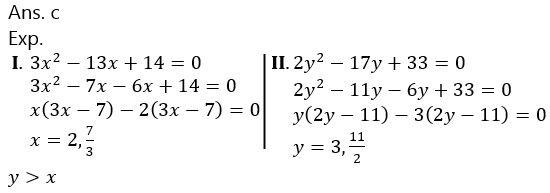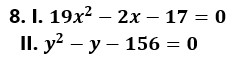(a)        if x>y

(b)        if x≥y

(c)         if x<y

(d)        if x ≤y

(e)        if x = y or  no relation can be established between x and y.(a)        if x>y

(b)        if x≥y

(c)         if x<y

(d)        if x ≤y

(e)        if x = y or  no relation can be established between x and y.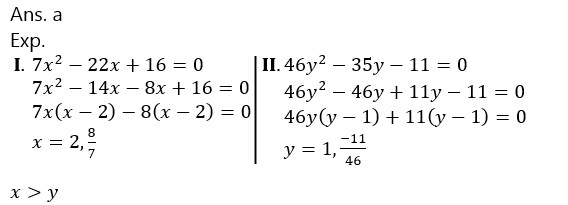(a)        if x>y

(b)        if x≥y

(c)         if x<y

(d)        if x ≤y

(e)        if x = y or  no relation can be established between x and y.### 2023 Preparation Kit PDF

#### Most important PDF’s for Bank, SSC, Railway and Other Government Exam : Download PDF Now

AATMA-NIRBHAR Series- Static GK/Awareness Practice Ebook PDF Get PDF here
The Banking Awareness 500 MCQs E-book| Bilingual (Hindi + English) Get PDF here
AATMA-NIRBHAR Series- Banking Awareness Practice Ebook PDF Get PDF here
Computer Awareness Capsule 2.O Get PDF here
AATMA-NIRBHAR Series Quantitative Aptitude Topic-Wise PDF 2020 Get PDF here
Memory Based Puzzle E-book | 2016-19 Exams Covered Get PDF here
Caselet Data Interpretation 200 Questions Get PDF here
Puzzle & Seating Arrangement E-Book for BANK PO MAINS (Vol-1) Get PDF here
ARITHMETIC DATA INTERPRETATION 2.O E-book Get PDF here

3